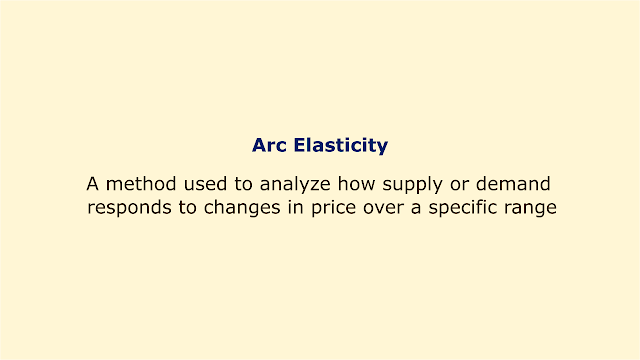# Arc ElasticityImage: Moneybestpal.com

### Arc elasticity is a technique used to examine how supply or demand reacts to changes in price across a particular range. The main goal of this study is to determine how sensitive producers or consumers are to changes in pricing.

Arc elasticity is distinct from point elasticity, which gauges the flexibility of a curve at a single point. By calculating the percentage changes in price and quantity using the midpoint formula, arc elasticity calculates the average elasticity over a range of values.

The formula for arc elasticity of demand is:

Arc elasticity of demand = (Q2 - Q1) / ((Q2 + Q1) / 2) / (P2 - P1) / ((P2 + P1) / 2)

Where:
• Q1 and Q2 are the initial and final quantities demanded
• P1 and P2 are the initial and final prices

The advantage of using arc elasticity is that it gives the same value for price increases and decreases of equal amounts. For example, if the price of a product increases from \$6 to \$8, and the quantity demanded decreases from 40 units to 20 units, the arc elasticity of demand is:

Arc elasticity of demand = (20 - 40) / ((20 + 40) / 2) / (8 - 6) / ((8 + 6) / 2)

Arc elasticity of demand = -0.5 / 0.25

Arc elasticity of demand = -2

If the price decreases from \$8 to \$6, and the quantity demanded increases from 20 units to 40 units, the arc elasticity of demand is:

Arc elasticity of demand = (40 - 20) / ((40 + 20) / 2) / (6 - 8) / ((6 + 8) / 2)

Arc elasticity of demand = 0.5 / -0.25

Arc elasticity of demand = -2

In both cases, the arc elasticity of demand is -2, indicating that the demand is elastic and responsive to price changes.

Arc elasticity is commonly used in economics to measure the inverse relationship between price and demand. It can also be used to measure the responsiveness of supply to price changes, using a similar formula:

Arc elasticity of supply = (Q2 - Q1) / ((Q2 + Q1) / 2) / (P2 - P1) / ((P2 + P1) / 2)

Where:
• Q1 and Q2 are the initial and final quantities supplied
• P1 and P2 are the initial and final prices

Arc elasticity can be used to determine a customer's willingness to pay and determine how much to charge for a product to maximize profitability. For instance, if a monopolist thinks that a product's demand is inelastic, they can set a high price to profit from the consumer's low sensitivity to price changes. In contrast, if demand is elastic, they might set a low price to boost sales volume and profits.

Arc elasticity can also be utilized in price discrimination, which is the practice of charging various prices to different clients for the same goods or services. An airline corporation could, for instance, charge higher rates for business passengers with inelastic demand for air travel and lower rates for leisure travelers with elastic demand.
Tags